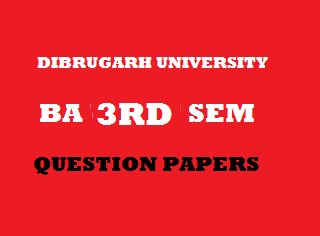## Thursday, January 03, 20192014
(November)
ECONOMICS
(General)
Course: 301
[Public Economics]
Full Marks: 80
Pass Marks: 32
Time: 3 hours
The figures in the margin indicate full marks for the questions

1. Choose the correct option/Answer the following/Fill in the blank: 1x8=8
1. Mention one similarity between public finance and private finance.
2. Which type of tax helps in reducing income disparities?
1. Proportionate tax.
2. Progressive tax.
3. Regressive tax.
4. All of the above.
1. What is horizontal equity in taxation?
2. Dalton classified public expenditure into two parts, grants and ____.
3. Write two limitations of fiscal policy.
4. Name two direct taxes levied by Government of India.
5. Name two items of non-plan expenditure of Indian Government.
6. Mention two functions of the Finance Commission.
2. Write short notes on any four of the following (within 150 words each): 4x4=16
1. Role of public finance in developed economy.
2. Objectives of taxation in developing country.
3. Merit goods.
4. Main elements of a budget.
5. Defects of Indian tax system.
Answer the following questions (within 500 words each):
3. (a) Define public finance. Discuss the difference between public finance and private finance. 2+9=11
Or
(b) Explain with diagram, the theory of maximum social advantage applied in the management of public finance. What are the limitations of the theory? 8+3=11

4. (a) Discuss the relative importance of direct and indirect taxes in a tax system. Explain which type of tax is more effective in achieving the following objectives:
1. Removal of income inequality.
2. Reduction in the price of cosmetic goods.
Or
(b) What do you understand by incidence of tax? Explain with diagram, how incidence of a tax is distributed between the buyers and the sellers. 2+9=11
5. (a) What are the main objectives of public expenditure? Give reasons for the rapid increase in public expenditure in a modern State. 6+6=12
Or
(b) What do you mean by burden of public debt? Discuss the methods of repayment of public debt.        4+8=12
6. (a) What is fiscal policy? What should be the objectives of fiscal policy in a developing economy?        3+8=11
Or
(b) What are the purposes of Government budget? Discuss different stages followed in the preparation of the budget. 4+7=11
7. (a) Analyze the main trends in the expenditure of the Government of India. Show the causes for the growth of such expenditure. 6+5=11
Or
(b) Write a note on India’s latest Union Budget. 11

***